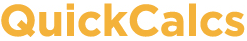1. Select category 2. Choose calculator 3. Enter data 4. View results

## Post test calculator

[Updated Dec 2004 to correctly deal with repeated measures ANOVA.]

After two-way (or other) Analysis of Variance (ANOVA), you often wish to perform post tests to compare individual pairs of groups. Some programs don't perform post tests at all. Others, like GraphPad Prism, perform post tests for commonly-used experimental designs, but not for every experimental design. This calculator performs any post tests between pairs of cells that you select. But note that this calculator does not perform the ANOVA calculations -- you must use another program for that. Learn more about post tests (with example) and how the calculations are performed .

What about repeated measures ANOVA? If your post test compares the same set of subjects at two different times, then follow the instructions below. If your post test compare two sets of subjects at one time, then the situation is trickier . For that post test, the fact that you also measured the same subjects at other times is irrelevant. So the MSresidual reported in the ANOVA table is not the right value to use for the post test. Instead, rerun the analysis as an ordinary two-way ANOVA and enter that MSresidual (and corresonding DF value) in the form below. Before spending much time on these post tests, consider whether two-way repeated-measures ANOVA is really the best analysis .

If you are running post tests at every dose along a dose-response curve, or every time point along a kinetic curve, think hard about what you really want to know. I don't think these post tests are very useful.

### 1. Enter ANOVA results

Find the row in the ANOVA table labeled 'residual' or 'error' (ordinary ANOVA) and enter the Mean Square (MS) and degrees of freedom (DF) below.
 MSresidual: DFresidual:

### 2. Choose alpha

Pick a significance level, alpha .
(0.05 is standard.)
Alpha = 0.01 (confidence level = 99%)
Alpha = 0.05 (confidence level = 95%)
Alpha = 0.10 (confidence level = 90%)
Alpha =

### 3. Enter the comparisons below

Enter one comparison in each row. For each comparison, enter the mean values for the two cells. Also enter sample size for each. Optionally enter labels to help you organize the results. This calculator compares pairs of cells; it does not compare entire rows or columns.

### 4. View the results

Label Mean1 Mean2 N1 N2
1:
2:
3:
4:
5:
6:
7:
8:
9:
10:
11:
12:
13:
14:
15:
16:
17:
18:
19:
20:
21:
22:
23:
24:
25:
26:
27:
28:
29:
30:
31:
32:
33:
34:
35:
36:
37:
38:
39:
40:
41:
42:
43:
44:
45:
46:
47:
48:
49:
50: